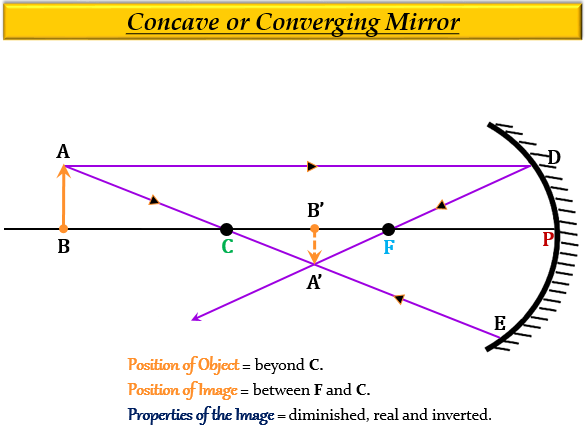# The real image formed by a concave mirror is smaller than the object if the object is:(a) between centre of curvature and focus (b) at a distance greater than radius of curvature (c) at a distance equal to radius of curvature (d) at a distance equal to focal length

(b) at a distance greater than the radius of curvature

Explanation

For an object placed beyond the centre of curvature $(C)$ or at a distance greater than the radius of curvature $(R)$, the image formed by a concave mirror is real and inverted, smaller than the object (diminished) and between focus $(F)$, and centre of curvature $(C)$.Updated on: 10-Oct-2022

126 Views